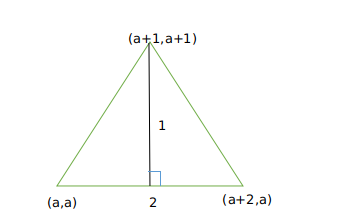Question 15

# The area of the triangle whose vertices are (a,a), (a + 1, a + 1) and (a + 2, a) is[CAT 2002]

Solution

The triangle we have is :The length of three sides is $$\sqrt 2, \sqrt 2$$ and $$2$$.
This is a right-angled triangle.
Hence, it's area equals $$1/2 * \sqrt 2 * \sqrt 2 = 1$$
So, the correct answer is b)
Alternate Approach :
Area of triangle = $$\frac{1}{2}\times\ base\times\ height$$
So we get $$\frac{1}{2}\times2\times\ 1$$=1 square units